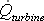# HOMER Pro 3.10

 Navigation: HOMER's Calculations How HOMER Calculates the Hydro Power OutputIn each time step, HOMER calculates the electrical power output of the Hydro turbine using the following equation:where: Phyd = power output of the hydro turbine [kW] ηhyd ρwater = density of water [1000 kg/m3] g = acceleration due to gravity [9.81 m/s2] hnet = effective head [m]3/s]